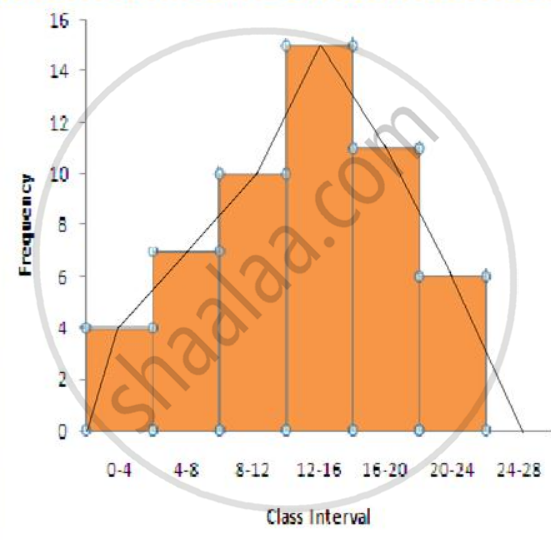# Construct a Frequency Polygon for the Following Distribution: - Mathematics

Graph
Sum

Construct a frequency polygon for the following distribution:

 Class-intervals 0-4 4 - 8 8 - 12 12 - 16 16 - 20 20 - 24 Frequency 4 7 10 15 11 6

#### Solution

The frequency polygon is shown in the following figureSteps:

(i) Drawing a histogram for the given data.

(ii) Marking the mid-point at the top of each rectangle of the histogram drawn.

(iii) Also, marking mid-point of the immediately lower class-interval and mid-point of the immediately higher class-interval.

(iv) Joining the consecutive mid-points marked by straight lines to obtain the required frequency polygon.

Concept: Graphical Representation of Data
Is there an error in this question or solution?

#### APPEARS IN

Selina Concise Mathematics Class 9 ICSE
Chapter 18 Statistics
Exercise 18 (B) | Q 1 | Page 233